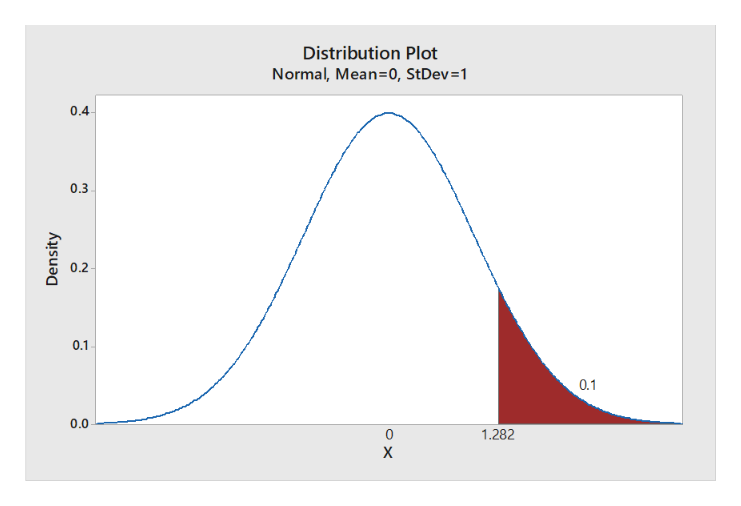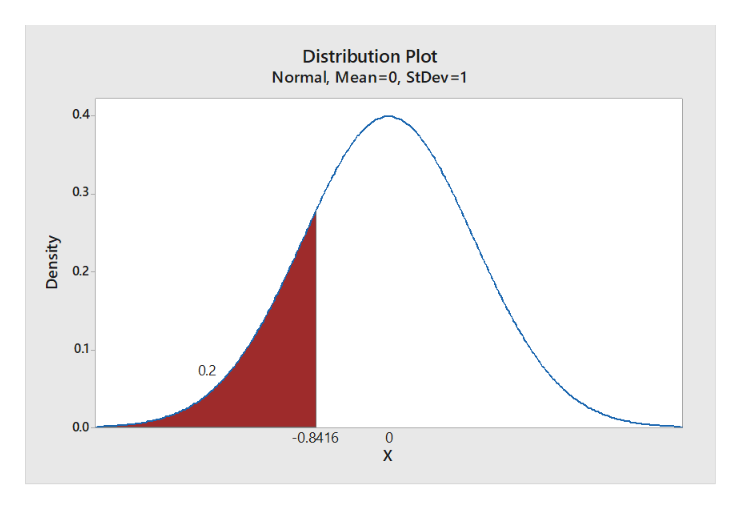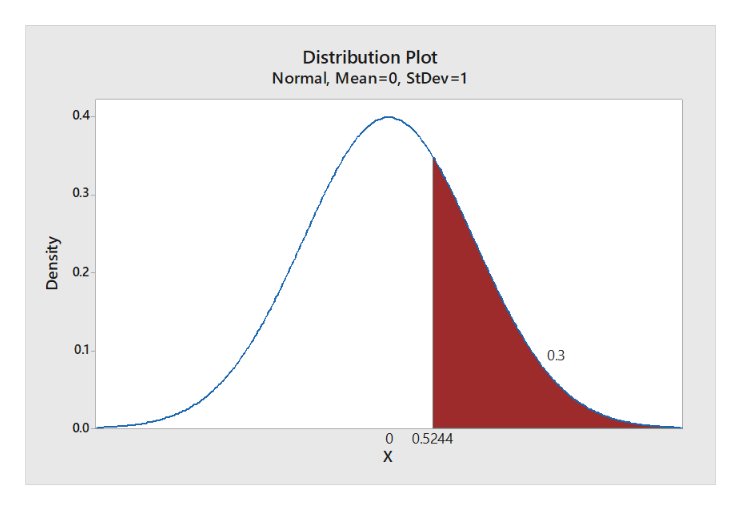# For a normal distribution, identify the z-score location that would separate the distribution into 2 sections so that there is10% in the tail on the right-hand side20% in the tail on the left-hand side30% in the tail on the right-hand side

Question
12 views

For a normal distribution, identify the z-score location that would separate the distribution into 2 sections so that there is

1. 10% in the tail on the right-hand side
2. 20% in the tail on the left-hand side
3. 30% in the tail on the right-hand side
check_circle

Step 1

1.

Step-by-step procedure to obtain the z-score location using MINITAB:

• Choose Graph > Probability Distribution Plot choose View Probability > OK.
• From Distribution, choose ‘Normal’ distribution.
• Choose Probability and Right Tail for the region of the curve to shade.
• Enter the Probability value as 10.
• Click OK.

Output using the MINITAB software is given below:help_outlineImage TranscriptioncloseDistribution Plot Normal, Mean-0, StDev=1 0.4 0.3 0.2 0.1 0.1 0.0 1.282 X Density fullscreen
Step 2

From the output, the z-score location that would separate the distribution into 2 sections so that there is 10% in the tail on the right-hand side is 1.282.

2.

Step-by-step procedure to obtain the z-score location using MINITAB:

• Choose Graph > Probability Distribution Plot choose View Probability > OK.
• From Distribution, choose ‘Normal’ distribution.
• Choose Probability and Left Tail for the region of the curve to shade.
• Enter the Probability value as 20.
• Click OK.

Output using the MINITAB software is given below:help_outlineImage TranscriptioncloseDistribution Plot Normal, Mean-0, StDev=1 0.4 0.3 0.2 0.1 0.2 0.0 -0.8416 0 X Density fullscreen
Step 3

From the output, the z-score location that would separate the distribution into 2 sections so that there is 20% in the tail on the Left-hand side is –0.8416.

3.

Step-by-step procedure to obtain the z-score location using MINITAB:

• Choose Graph > Probability Distribution Plot choose View Probability > OK.
• From Distribution, choose ‘Normal’ distribution.
• Choose Probability...help_outlineImage TranscriptioncloseDistribution Plot Normal, Mean=0, StDev=1 0.4 0.3 0.2 0.1 0.3 0.0 0 0.5244 х Density O fullscreen

### Want to see the full answer?

See Solution

#### Want to see this answer and more?

Solutions are written by subject experts who are available 24/7. Questions are typically answered within 1 hour.*

See Solution
*Response times may vary by subject and question.
Tagged in

### Other